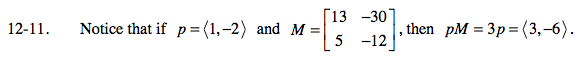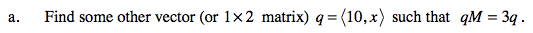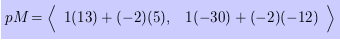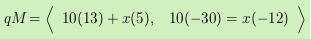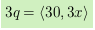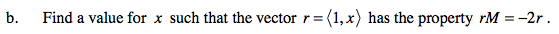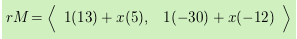### Home > PC > Chapter 12 > Lesson 12.1.1 > Problem12-11

12-11.
1. Notice that if p = (1, −2) and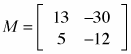, then pM = 3p = (3, −6). Homework Help ✎

1. Find some other vector (or 1 × 2 matrix) q =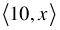such that qM = 3q.

2. Find a value for x such that the vector r =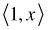has the property rM = −2r.